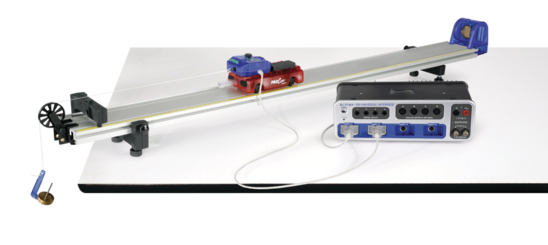# Oscillation Equations of Motion

The motion of an oscillating mass is measured using a Motion Sensor, and graphs are produced of position, velocity and acceleration. The relative phase of each is examined, and compared to theory. Calculations are made for the maximum velocity and maximum acceleration during an oscillation and the equation of motion is written for this system.

Student Files

 43_Oscillation_Equations_of_Motion_w_Data.cap 534.26 KB 43_Oscillation_Equations_of_Motion.cap 499.61 KB 43_Oscillation_Equations_of_Motion.docx 581.88 KB 43_String.jpg 183.59 KB 43_Mass_and_Spring.jpg 358.33 KB 43_Oscillation_Equations_of_Motion.pdf 107.82 KB
Source: Lab #43

## Comprehensive 850 Physics System